Box-Cox Transforms and Other Nonparametric Normalization MethodsSo that is a bit of a snarky title. Today, I want to talk about techniques that you can use to transform non-normal data into a distribution that makes way more sense. This can be useful for all kinds of analysis like time-series I deal with non-normal data a lot. In fact, I’ve come to the opinion that the most normal thing about data is that it usually isn’t normally distributed. However, in many cases we would like to use a test that requires normality. I’ve seen a number of solutions that normalize data so that it fits on a certain scale, like zero to one, or data that is scaled in a certain way so that your variable is expressed in interesting units, like standard deviation units. I’ve even seen non-linear transformations like box-cox transformations which try to use a parametric function to cram the data into a normal distribution, more on this technique later.

Generally, what I see when I see somebody normalize their data is that they take the data and they transform it with a linear transformation. A good example is to scale the data so that it falls on the zero to one scale. This requires knowledge of the minimum and maximum for the data, but once you know that, you can make a linear transformation. You can verify this by taking the pearson correlation coefficient between the rescaled data and the original. You will get a correlation coefficient of one. That tells you that fundamentally, you haven’t done any alteration to the data. You can also convert the data into z-scores, this requires knowledge of the mean and standard deviation, but it is essentially a linear transformation. Again this does not fundamentally change the nature of your data, and you will get a correlation coefficient of unity. So the distribution doesn’t change using these methods, only the scale. And in certain circumstances, that is fine. Neural networks, for example, seem to like having all of the data on the same scale as the output. So making a linear transformation makes sense.

The Box-Cox Transform

Sometimes, however, we want to have nice bell curve shaped data, or maybe, we want a nice uniform distribution to the data because of some statistical test that we want to run. But data is so rarely well-behaved. If you have read up on statistics you might know something about the box-cox transform. This nifty little function has a single parameter. Which when that parameter hits a magic value, specific to your dataset, it will transform your data the best that it can to look as bell curve like that it can. That’s all well and good, except that there is a problem. Sometimes it does a great job, and other times it kind of sucks.

Here’s the formula:Then you can use a maximum likelihood calculation using the normal distribution as your likelihood distribution to calculate the correct value of lambda. The only problem is that, now you have inherently provided extra uncertainty into the test that you are going to conduct. It pains me when I see this, because, that extra uncertainty is rarely, if never accounted for in the test. But I digress as that would be a potentially fun statistics paper to write for an academic journal, and that isn’t what I set out to do in this post.

Helpfully, the stats module in scipy has a nice function for doing a box-cox transform. It will even do that pesky maximum likelihood business for you. Even though I don’t like that the extra uncertainty isn’t accounted for very well in the statistical tests, the box-cox transform is battle tested. It just seems to work. And as an economist, it holds a special place in my heart, because it is tightly connected to CES (constant elasticity of substitution) production/utility functions. I won’t beat this point home too hard for those of you that don’t want to geek out on the neat microeconomic implications of the box-cox transform, but for those of you that care, it is the inverse of the CES equation.

Anyway, like I mentioned earlier, the box-cox transformation doesn’t have a guarantee that you will end up with anything that has a distribution that has the same properties as a normal distribution. It just says that it will find the best approximation constrained to that functional form. Usually, it does pretty good. Sometimes, it fails spectacularly. Either way, it works by making a monotonic transformation of the data.

Detour: What is a monontonic transformation?

Great question, a monotonic transformation is any transformation that never changes the direction, but only the magnitude of its slope.

I saw eyes glaze over. Let me try again, for our purposes, a monotonic function is a function that is always increasing. In other words, the derivative is always positive. Okay, so it could also be that it’s derivative is always negative, but just multiply everything by -1, and then you get a function that is always increasing. Use that as your working definition of what a monotonic transformation is. The technical definition is a little more complicated, but this is the definition that I carry around in my head.

Your next question is probably why do we care about monotonic transformations? Well, we like them because they do not change the ordering of our data. In microeconomics, we like them because utility functions don’t have meaningful units, so we can do a monotonic transformation to make the calculus simpler. For statistics, it means that our data will still reflect reality (the ordering of the variables stays correct, but the spacing might not.) It will screw up the units we are working with and make the interpretation of a test, coefficient, etc. harder for us in the end. Although, we may gain more than we lose, or lose more than we gain, depending on what we want to do.

The problem with box-cox transforms is that they are parametric, which gives them constraints. For the rest of this post, I will consider two non-parametric approaches to normalizing the data in such a way that we force the data to have a certain distribution. That might be useful, for example, in eliminating human bias from a dataset that relies on human judgment, like movie ratings, beauty contest scores, or figure skating competition scores. That’s right, I’m pulling the winter Olympics into the blog post.

So I grabbed the scores for the top 24 female figure skaters in the 2014 Sochi games. The reason for top 24 is that those are qualifying athletes that got to participate in the full competition. Although we could add a correction to the truncated scores I didn’t want to over complicate things. The scores ranged from a high of 224.59 and a low of 125 points.

Let’s take a look at the distribution of those points.

df.dropna(subset=['Rank'],inplace=True)
df.index = range(len(df))
df['Result'].hist()
plt.title("Distribution of Women's Figure Skating Scores"
"\nSochi 2014 Raw Scores")
plt.ylabel('Number of Athletes')
plt.xlabel('Score')
plt.show()

Which will produce this figure: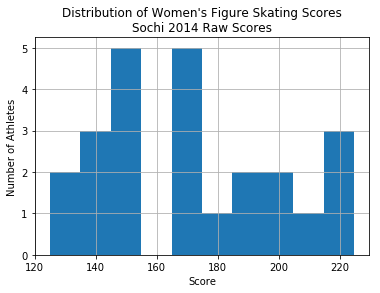We would expect something that looks normally distributed. Especially, since we are dealing with human levels of achievement. You would expect to see a bell curve around Olympic quality figure skating. But we can do a non-parametric transformation of this data to force it into a specific distribution. For example, let’s say that I want to force a uniform distribution on the data. I can use percentile normalization. Here’s the code:

def percentile_norm(data):
mem_cache = {}
out_list = []
for i in data:
if i not in mem_cache:
mem_cache[i] = percentileofscore(data, i, kind='strict') / 100.0
out_list.append(mem_cache[i])
return out_list

This code uses a memory caching technique to speed up the computations. Other than that it simply maps the percentile of a data point to the data point. It is a monotonic transformation because it preserves the ordering of the data. If you plot the distribution of this data you can confirm that you have morphed the distribution into a uniform distribution.

df['pnorm'] = percentile_norm(df['Result'])
plt.hist(df['pnorm'])
plt.title("Distribution of Women's Figure Skating Scores"
"\nSochi 2014 Percentile Normalized")
plt.xlabel('Normalized Score')
plt.ylabel('Number of Athletes')
plt.show()

Which will produce this histogram: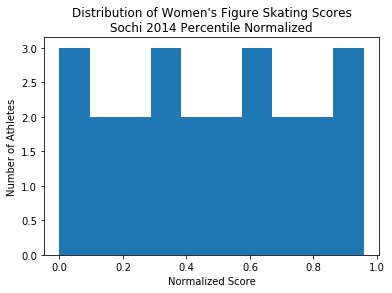So yeah it’s uniform. And if you want to make sure that we really did do a monotonic transformation of the data, you can plot the original values against the normalized values.

plt.scatter(df['Result'],df['pnorm'])
plt.title('Monotonic Transformation for Percentile Normalization')
plt.show()

Which gives you this figure: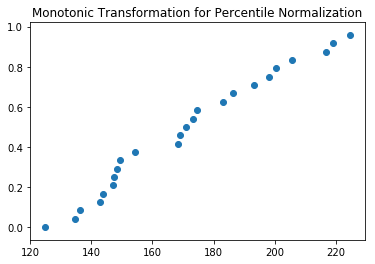Notice that it isn’t exactly a nice looking function, that would be easy to parameterize, although I suppose you could do it. This method is non-parametric, a
as opposed to the box-cox transformation.

But we didn’t want a uniform distribution, we wanted to have a nice gaussian distribution. That is where gaussian quantile normalization comes in. The code below implements it using the same memory caching technique that we used for percentile normalization. Notice, that I kind of cheat by taking a random sample. You could fix this by looking up values for the percentile normalized scores off of the inverse cummulative distribution function, but hey, I was getting lazy. So make sure to set your seed or results may vary.

def gaussian_quantile_norm(data, seed=None):
if seed is not None:
np.random.seed(seed)
gauss = norm.rvs(size=len(data))
gauss = np.sort(gauss)
test = np.sort(data)
mem_cache = {}
out_list = []
for i in range(len(data)):
if test[i] not in mem_cache:
mem_cache[test[i]] = gauss[i]
for i in range(len(data)):
out_list.append(mem_cache[data[i]])
return out_list

I won’t beat the drum of how to create analogous graphs to those that I did for the percentile normalization. I think you should be able to figure that out. Or just go to my github repo and grab the code for this tutorial. So here is the figures for gaussian quantile normalization: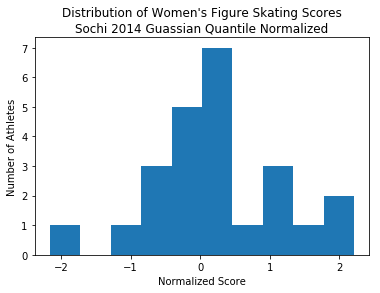Also using the scipy implementation of the box-cox transform this is what you get.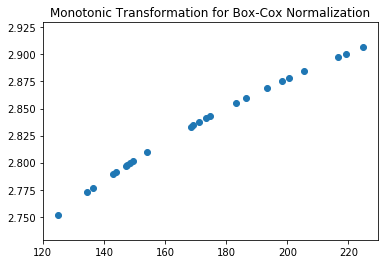Notice that the Box-Cox Transform is an almost linear function. That would say that our data was already mostly bell-curve shaped. So we didn’t need to do that. Also we can check the hypothesis that the lambda value from the equation above is statistically significant. If it isn’t then we can say that the data is approximately normally distributed. Which I’m not going to do, because well, I’m tired. But give it a try.